• ## R语言 绘制散点图

万次阅读 2018-08-27 11:43:39
简单地说，把一些R语句（赋值、计算或其他操作步骤）包 装起来并给它一个名称，这就是函数。我们前面接触过的 getClass( ), class( ), head( ), rep( ), cbind( ), rbind( )等都是函数。显示（打印）对象...
［转自：http://blog.sina.com.cn/s/blog_69ffa1f90101siek.html］
函数。简单地说，把一些R语句（赋值、计算或其他操作步骤）包 装起来并给它一个名称，这就是函数。我们前面接触过的 getClass( ), class( ), head( ), rep( ), cbind( ), rbind( )等都是函数。显示（打印）对象 也有函数print( )，但R有更简单的方法：输入对象名（数据对象或函数名）就可以显示其内容。函数一般都有参数。
R 作图也是通过函数完成的，不同函数可以做出不同的图形，比如plot()函数可以做散点图、线状图，barplot()函数可以做出柱状图或条形 图，pie()函数用于做饼形图。作图或者称数据可视化，是R的主要的功能之一。R语言虽然是一种计算机语言，但用R作图不一定要会编程，相当简单。
散点图是最基本的数据分析图形，我们就从它开始。
一、文件数据读取和保存
作图首先要有数据。为避免重复输入，我们一般将R使用的数据存成文本文件（txt文件）或逗号分隔符文件（csv文件），Excel里面有这样的保存选项。
好的R软件包一般都提供有一些供学习和举例用的样本数据，这些数据可以用data( )函数来查询。为方便说明，我们使用R（base包）提供的样本数据。先看看有些什么：

> data()
Data sets in package 'datasets':

AirPassengers           Monthly Airline Passenger Numbers 1949-1960
BJsales                 Sales Data with Leading Indicator
BOD                     Biochemical Oxygen Demand
CO2                     Carbon Dioxide Uptake in Grass Plants
ChickWeight             Weight versus age of chicks on different diets
DNase                   Elisa assay of DNase

#...........（还有很多，节省篇幅不列了）
我们用trees数据来做散点图。先看看它是什么样的数据：

> str(trees)     #数据的结构
'data.frame':    31 obs. of  3 variables:
$Girth : num 8.3 8.6 8.8 10.5 10.7 10.8 11 11 11.1 11.2 ...$ Height: num  70 65 63 72 81 83 66 75 80 75 ...
$Volume: num 10.3 10.3 10.2 16.4 18.8 19.7 15.6 18.2 22.6 19.9 ... > head(trees) #数据的前几条记录 Girth Height Volume 1 8.3 70 10.3 2 8.6 65 10.3 3 8.8 63 10.2 4 10.5 72 16.4 5 10.7 81 18.8 6 10.8 83 19.7 可以看到trees数据集是我们比较熟悉的数据框类型，有3个列变量。我们把它保存到D盘根目录。首先用setwd( )函数设置工作目录，它是R读取和保存文件的默认位置。注意：如果是反斜杠得用两个。下面两条语句效果都一样： > setwd("d:/") > setwd("d:\\") 然后用write.csv( )函数保存成trees.csv文件： > write.csv(trees, "trees.csv") 用read.csv( )函数读取数据到the.data中： > the.data <- read.csv("trees.csv", header = TRUE) 再看看读入的数据是什么样子： > str(the.data) 'data.frame': 31 obs. of 4 variables:$ X     : int  1 2 3 4 5 6 7 8 9 10 ...
$Girth : num 8.3 8.6 8.8 10.5 10.7 10.8 11 11 11.1 11.2 ...$ Height: int  70 65 63 72 81 83 66 75 80 75 ...
$Volume: num 10.3 10.3 10.2 16.4 18.8 19.7 15.6 18.2 22.6 19.9 ... > head(the.data) X Girth Height Volume 1 1 8.3 70 10.3 2 2 8.6 65 10.3 3 3 8.8 63 10.2 4 4 10.5 72 16.4 5 5 10.7 81 18.8 6 6 10.8 83 19.7 read.csv函数读入的数据是数据框，多了表示序号的一列，不影响。如果嫌碍眼就去掉它。 > the.data <- the.data[ ,2:ncol(the.data)] 二、快速作图法 用plot函数可以非常便捷地做出散点图： > x <- the.data$Girth
> y <- the.data$Height > plot(x, y, main = "树围与树高关系图", xlab = "Girth", ylab = "Height")R的快速作图方式主要是针对数据关系的探索性分析的，不同类型的图完全可以显示出数据间的关系，同时避免了浪费时间在图形外观的设置上。如果想美化图形，也很容易。图形元素无非包括标题、坐标轴、点、线、图例等几个部分，外观包括形状、颜色、大小、宽度和字体等。 1、标题文字设置参数：main（主标题），sub（副标题），xlab（x轴标题）和ylab（y轴标题） 2、坐标轴有无：axes （TRUE/FALSE） 3、坐标轴区间参数：xlim，ylim 4、点类型参数：pch 5、线类型、宽度参数：lty，lwd 6、颜色设置参数：col, col.axis, col.lab, col.main, col.sub 7、大小（包括图形和字体）参数：cex, cex.axis, cex.main, cex.sub, cex.lab 8、如果点类型是空心点（pch=21-25），还可以设置内部填充颜色：bg参数 我们通过设置plot函数的参数做一个散点图： > plot(x, y, main = "树围与树高关系图", xlab = "Girth", ylab = "Height", xlim = c(0,25), #设置x轴刻度范围 pch = 2, col = "red", cex = 1.2, #设置图形几何形状和颜色 col.lab = "blue", col.axis = "orange", cex.lab = 1.2, cex.main = 1.5)为了避免输入错误后重复打字，一般先把绘图语句写到文本文件，然后拷贝到R里面就可以了。有关的文本编辑器后面再推荐和介绍，现在可以先用自己熟悉的任何文本编辑器做这个事情。 三、分步作图法 上面的图形可以一步步进行。 1、先绘制只设置了点的图，轴不显示，坐标标题为空： > plot(x, y, pch = 2, col = "red", axes =FALSE, xlab="", ylab="")2、添加其他图形元素（注意每执行一条语句后图形会马上有变化，中间过程的图形就不一一贴出了）： > plot(x, y, pch = 6, col = "red", axes =FALSE, xlab="", ylab="", xlim=c(8,22), ylim=c(10,90)) > box(col="green") > axis(1, col="orange", lty=2, lwd=3) > axis(2, col="purple", col.axis="purple") > axis(4, col="seagreen") > title(main="树围与树高关系图", cex.main = 1.5) > title(xlab="Girth", cex.lab=1.2, col.lab="blue")可以看到，分步作图法可以获得对图形元素的更多控制，各个坐标轴、标签的颜色和字体大小都可以单独设置。还可以在上面图形的基础上添加其他的数据点（在前面作图时注意设置y轴的范围）： > y2 <- the.data$Volume
> points(x, y2, pch=25, col="red", bg="blue")
> title(ylab="Height or Volume", cex.lab=1.2, col.lab="red")注 意：plot( )函数执行后会把图形区的其他东西全“擦”掉再新作图，这样的图形函数称为高水平图形函数；而 box( ), title( ), axis( ), points( )这些函数只是在原有图形基础上添加东西，它们称为低水平图形函数。只有使用了 高级图形函数产生图形区后才能使用低水平图形函数。各图形元素的低水平图形函数有：
1、添加标题（主、副标题和坐标轴标题）：title( )
2、添加坐标轴：axis( )
3、添加数据点：points( )
4、添加线：lines( )
5、添加水平线或垂直线：abline( )
6、其他text( ), polygon( ), legend( )在后面介绍。
四、R base函数作图的特点
以上方法是用都是R基本绘图包（graphics包，已包含在R基本安装包里面）带的作图函数来做的，这个过程就像拿起画笔在一张白纸上画画，每个图形元素都可以自己去设计。因此R graphics包作图的特点也就体现出来了：R提供了丰富的图元控制函数和参数，可以方便地描绘出所需的每个元素。但是和我们普通作图不一样的是，R作图没有橡皮擦，做错了只能重新来过。但这应该不是什么问题，R作图是用语句来进行的，重用这些语句可以绘制出完全一样的图，这点正是科学绘图所需要的。

但是，R把图形元素的控制全交给了我们，虽然很自由，是不是也很麻烦？这恐怕也是很多人学R作图过程中退却的原因吧？但这些设置是有懒办法的：把常用的作图参数设置好并作为函数封装起来（类似与一些软件的宏操作），这样就可以一劳永逸了。《用R语言绘制y轴不连续的柱形图》中自编的gap.bar函数就是这样的一个例子，函数自定义的方法请参考《R语言基础教程6：程序设计基础》部分。

展开全文• 使用R语言高级绘图函数对金融领域的数据进行绘图并做简单分析。 数据：金融案例数据 获取数据： getwd() setwd('D:\\') FinRatio <- read.csv("financial ratio.csv", header= T,stringsAsFactors=F, na.strin....
任务： 使用R语言高级绘图函数对金融领域的数据进行绘图并做简单分析。 R语言高级图形函数用的参数
数据：金融案例数据 获取数据：
getwd()
setwd('D:\\')
na.strings= "")#加载文件

数据处理：
FinRatio$Debt.to.Assets.Ratio <- as.numeric(FinRatio$Debt.to.Assets.Ratio,
na.rm=T) #移除na数据
FinRatio$ROA <- as.numeric(FinRatio$ROA,na.rm=T)#移除na数据
FinRatio$Total.Assets.Turnover <- as.numeric(FinRatio$Total.Assets.Turnover,
na.rm=T) #移除na数据
FinRatio$Cash.Ratio <- as.numeric(FinRatio$Cash.Ratio,
na.rm=T) #移除na数据

#1）多组散点图,分为 st 和非 st 上市公司
FinRatio$group <- as.factor(FinRatio$group)#转换成因子类型
par(mfcol = c(1,1))
# par(mforw=c(3,2))
# 则是在同一绘图区中绘制3行2列共6个图形,而且是先按行绘制，
#即绘制完第1行的2个图形后，再绘制第2行的2个图形，最后是第3行的2个图形。
# par(mfcol=c(3,2))
# 也是绘制3行2列共6个图形，与上面不同的是，先按列绘制。
#即先绘制完第1列的3个图形，再绘制第2列的3个图形。
FinRatio$a<-runif(2780,min=0,max=1) #runif(n,min=0,max=1)--n表示生成的随机数数量，min表示均匀分布的下限， #max表示均匀分布的上限；若省略参数min、max,则默认生成[0,1]上的均匀分布随机数。 m <-FinRatio[order(FinRatio$a),]
#order返回的是一个permutation。
#也就是说，把数从小到大排序的话，当前位置的数会排在第几个。
#plot(m$Debt.to.Assets.Ratio,m$ROA.,pch=as.integer(m$group),col=c(1:4)) plot(m$Debt.to.Assets.Ratio,m$ROA.,pch=as.integer(m$group))
# as.integer，将数据特别标明是integer类型。
# integer类型的数据主要是为了存储和计算更省空间,以及方便和其他语言保持一致
#pch缺省下设定数据显示为点状。pch 符号可以使用0 : 25来表示26 个标识
#如pch=23设定数据点显示形状为菱形；当pch=0时不显示任何符号；

运行结果：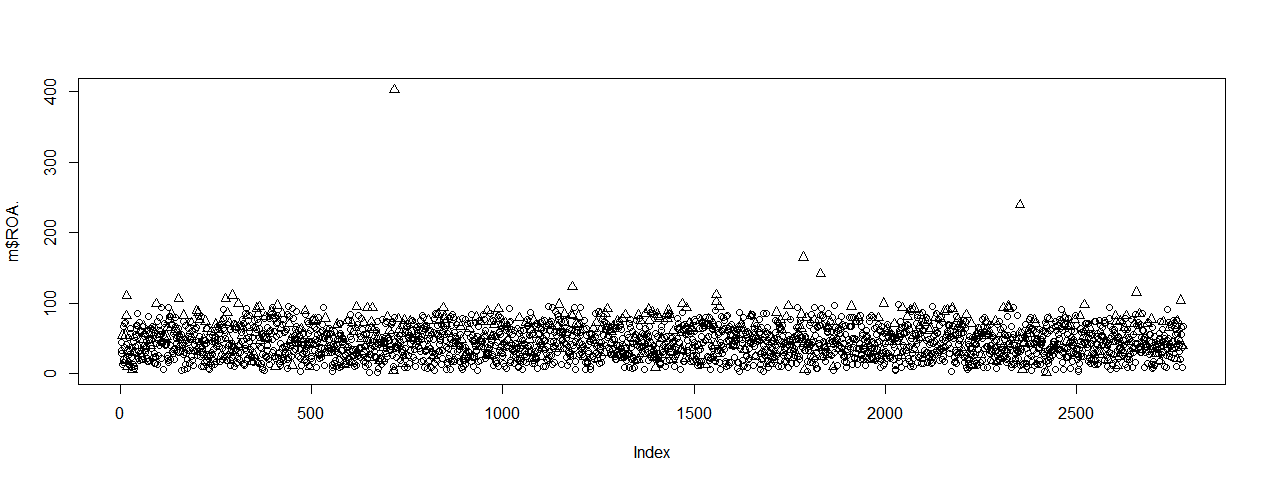本来想让st 公司一个颜色和非 st 上市公司为另一个颜色，然而莫有做到。。
plot(m$Debt.to.Assets.Ratio,m$ROA.,pch=as.integer(m$group),col=c(1:26))  运行结果：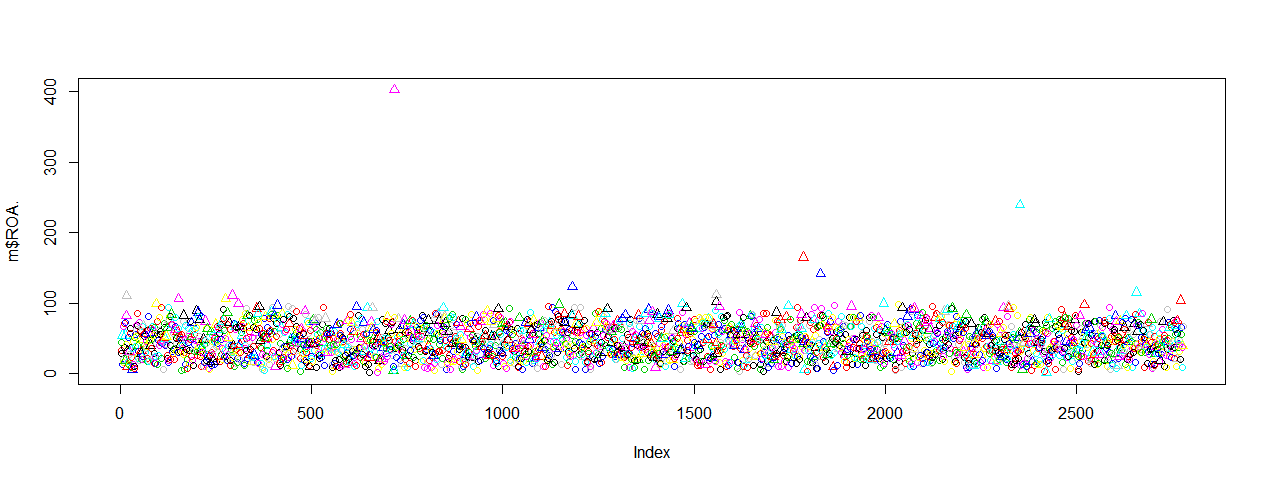#2) 多变量散点图 #head(FinRatio) #head(dataframe)——查看数据集前6行数据 plot(FinRatio[,1:4],col=c(1:26))  运行结果：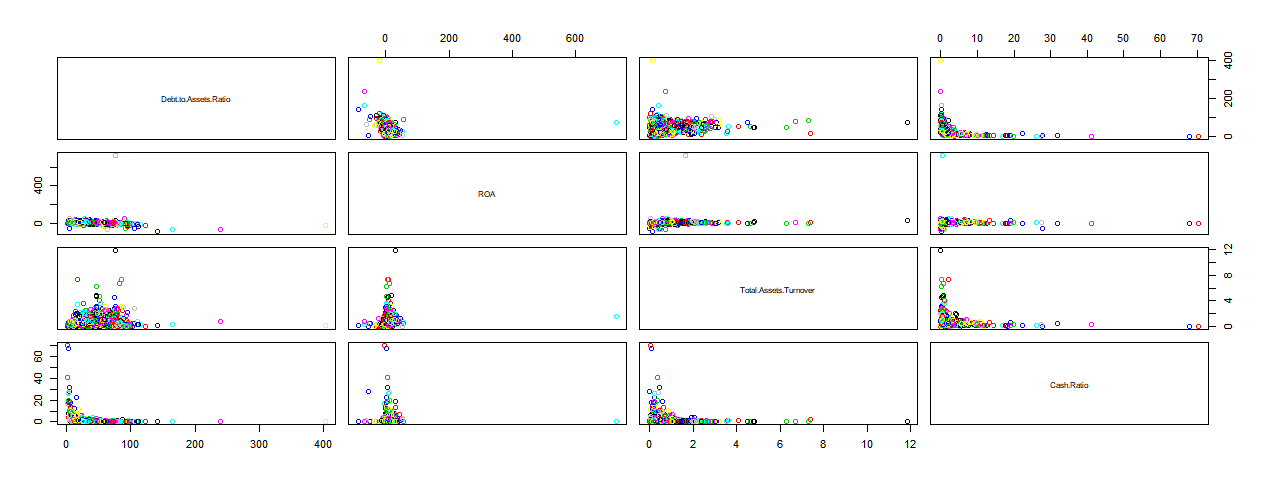#3)散点图+平滑线 plot(m$Debt.to.Assets.Ratio,m$ROA.) lines(lowess(m$Debt.to.Assets.Ratio,m$ROA.),col='red',lwd=3) #lowess--局部加权回归散点平滑算法。  运行结果：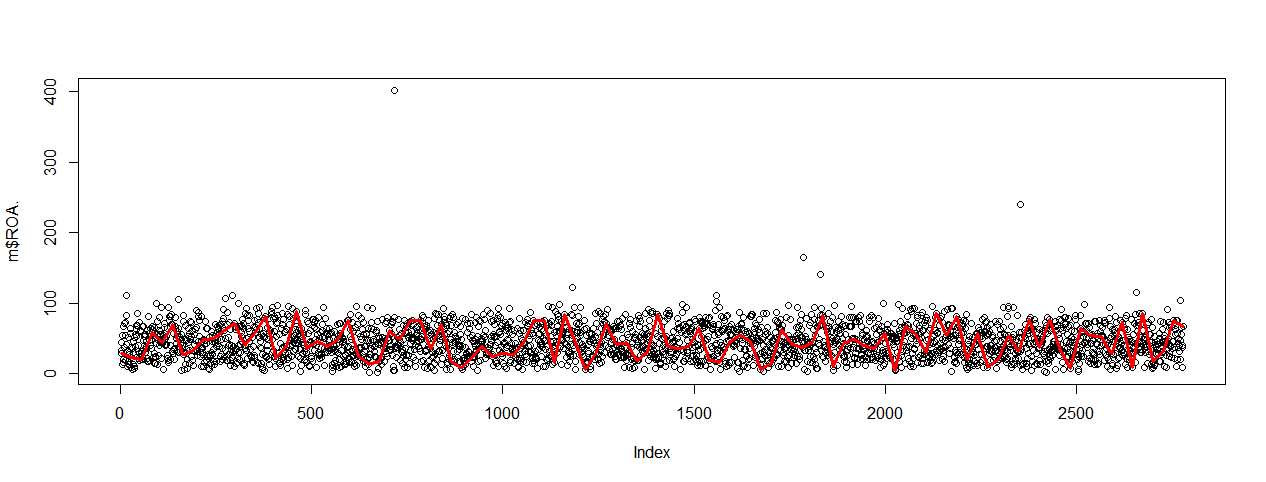#4)箱线图 FinRatio1 <- FinRatio[complete.cases(FinRatio),] plot(FinRatio1[,5],FinRatio1$Debt.to.Assets.Ratio,type='l', xlab="group", ylab="Debt.to.Assets.Ratio")

运行结果：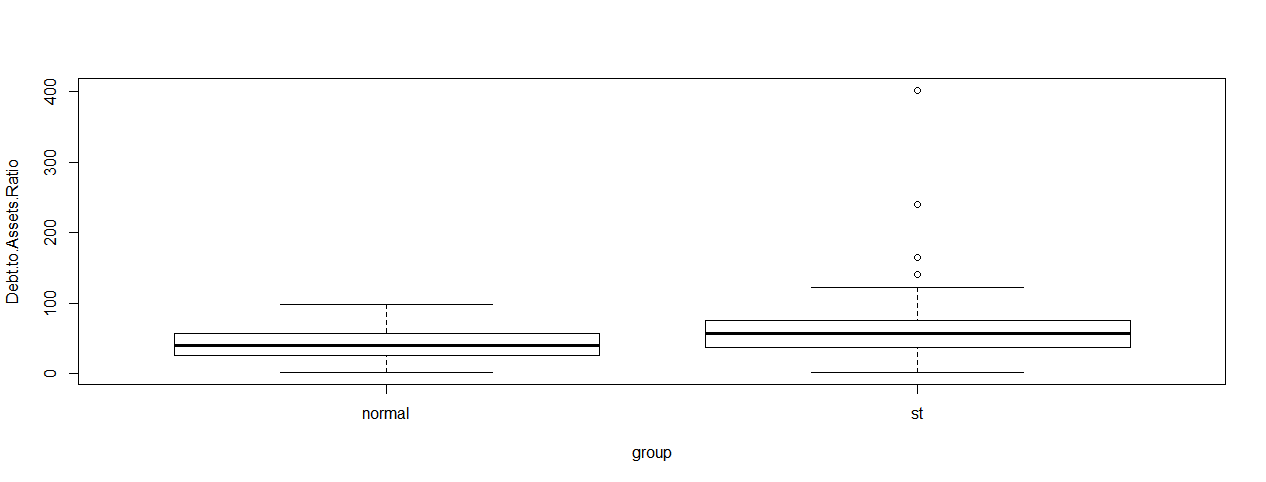plot(FinRatio1[,5],FinRatio1$ROA,type='l',xlab="group", ylab="ROA")  运行结果：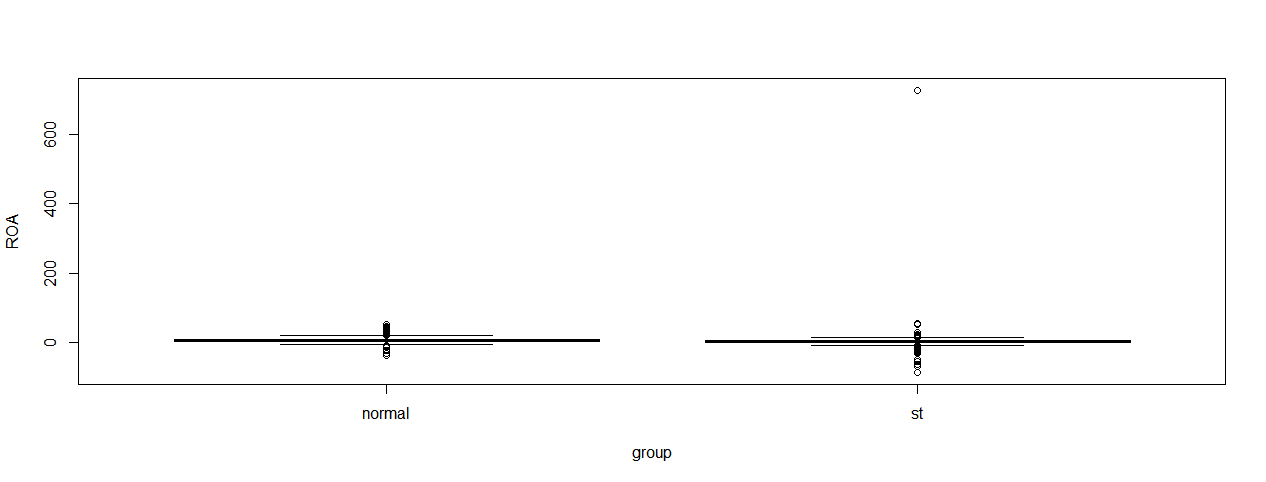plot(FinRatio1[,5],FinRatio1$Total.Assets.Turnover,type='l',xlab="group", ylab="Total.Assets.Turnover")

运行结果：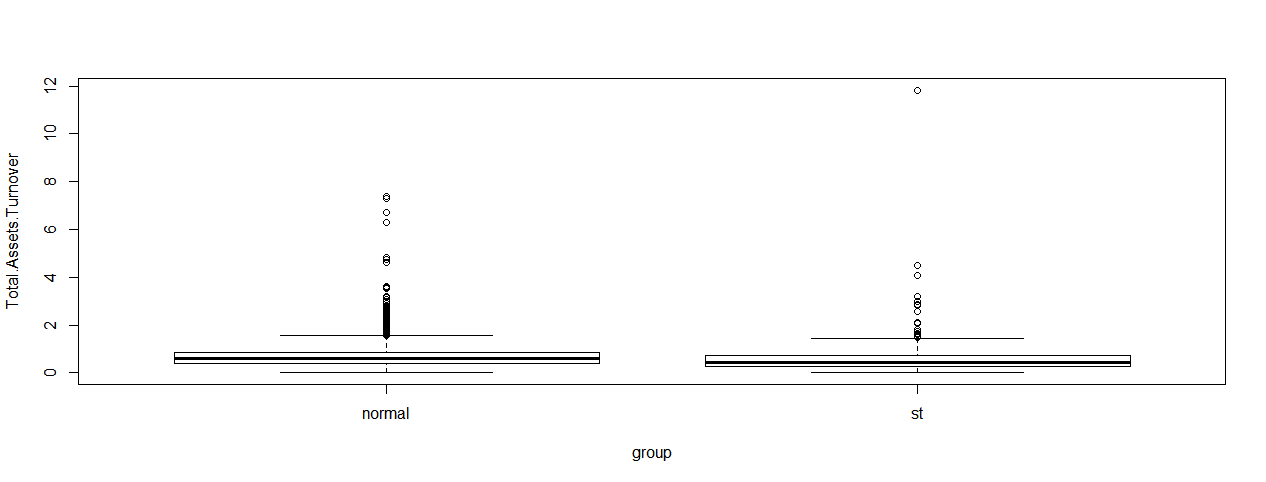plot(FinRatio1[,5],FinRatio1$Cash.Ratio,type='l',xlab="group", ylab="Cash.Ratio")  运行结果：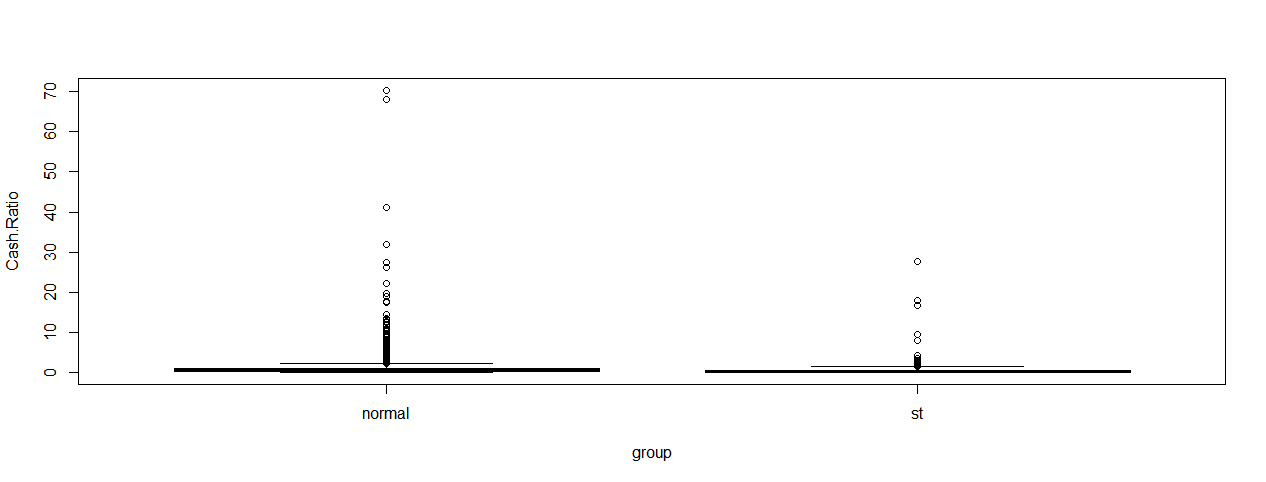展开全文• ## R语言中的高级绘图 万次阅读 2016-02-25 18:05:51 1、散点图 attach(mtcars) plot(wt, mpg, main="Basic Scatterplot of MPG vs. Weight", xlab="Car Weight (lbs/1000)", ylab="Mil 1、散点图 attach(mtcars) plot(wt, mpg, main="Basic Scatterplot of MPG vs. Weight", xlab="Car Weight (lbs/1000)", ylab="Miles Per Gallon ", pch=19) abline(lm(mpg ~ wt), col="red", lwd=2, lty=1) lines(lowess(wt, mpg), col="blue", lwd=2, lty=2) abline()函数用来添加最佳拟合的线性直线， lowess()函数则用来添加一条平滑曲线。该平滑曲线拟合是一种基于局部加权多项式回归的非参数方法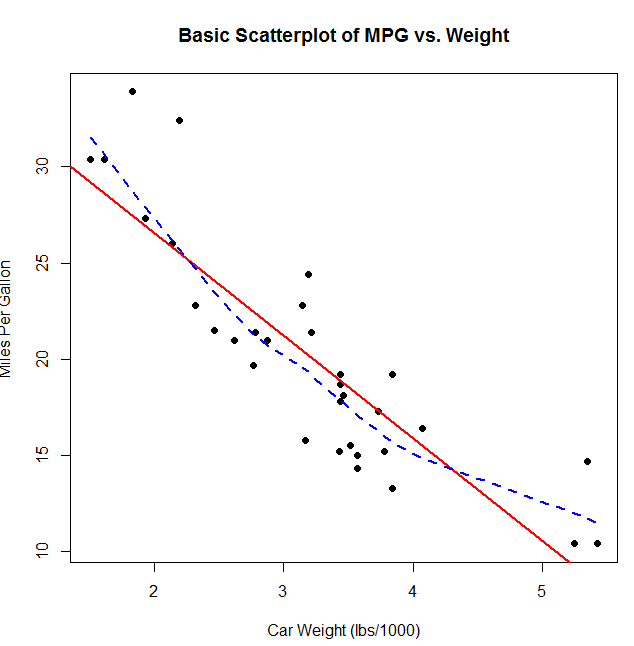library(car) scatterplot(mpg ~ wt | cyl, data=mtcars, lwd=2, main="Scatter Plot of MPG vs. Weight by # Cylinders", xlab="Weight of Car (lbs/1000)", ylab="Miles Per Gallon", id.method="identify", legend.plot=TRUE, labels=row.names(mtcars), boxplots="xy")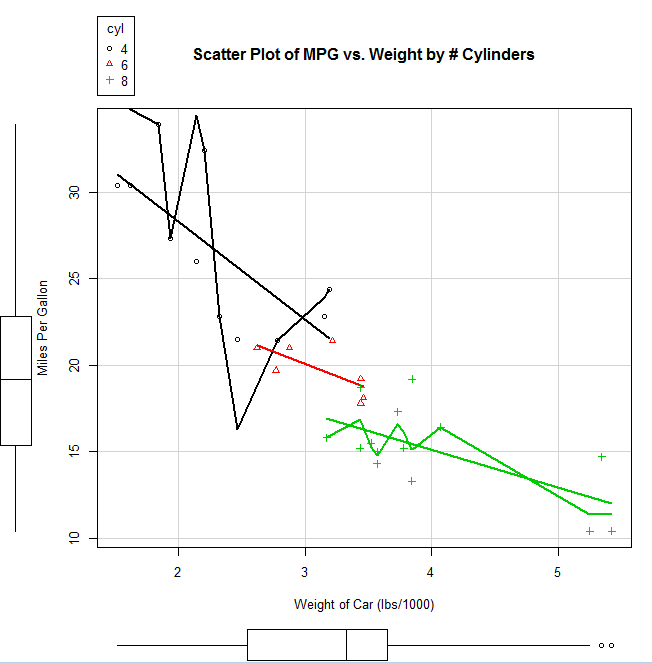2、散点图矩阵 （1）library(car) scatterplotMatrix(~ mpg + disp + drat + wt, data=mtcars, spread=FALSE, lty.smooth=2, main="Scatterplot Matrix via car package")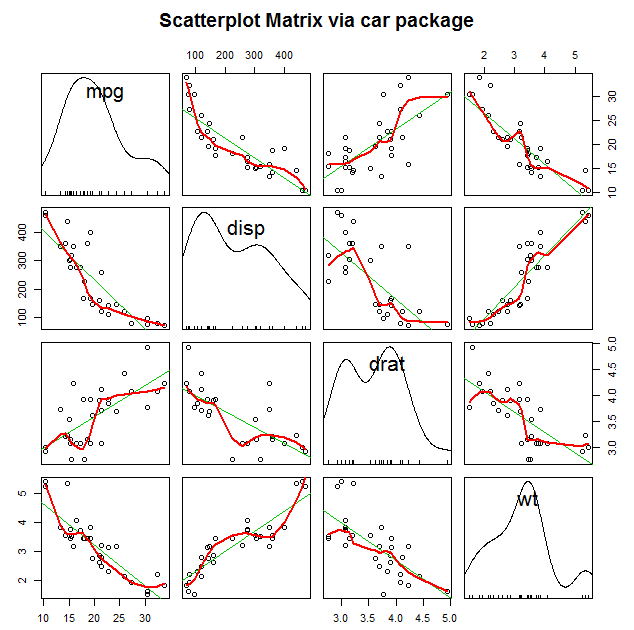scatterplotMatrix(~ mpg + disp + drat + wt | cyl, data=mtcars, spread=FALSE, main="Scatterplot Matrix via car package", diagonal="histogram") 以不同的气缸进行分组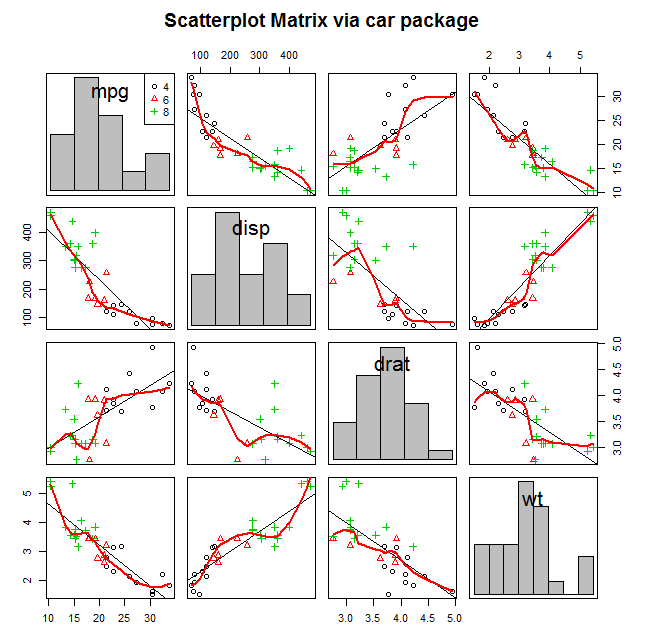cor(mtcars[c("mpg", "wt", "disp", "drat")]) mpg wt disp drat mpg 1.0000000 -0.8676594 -0.8475514 0.6811719 wt -0.8676594 1.0000000 0.8879799 -0.7124406 disp -0.8475514 0.8879799 1.0000000 -0.7102139 drat 0.6811719 -0.7124406 -0.7102139 1.0000000 使用gclus包绘制散点图矩阵 library(gclus) mydata <- mtcars[c(1,3,5,6)] mydata.corr <- abs(cor(mydata)) mycolors <- dmat.color(mydata.corr) myorder <- order.single(mydata.corr) cpairs(mydata, myorder, panel.colors=mycolors, gap=.5, main="Variables Ordered and Colored by Correlation" )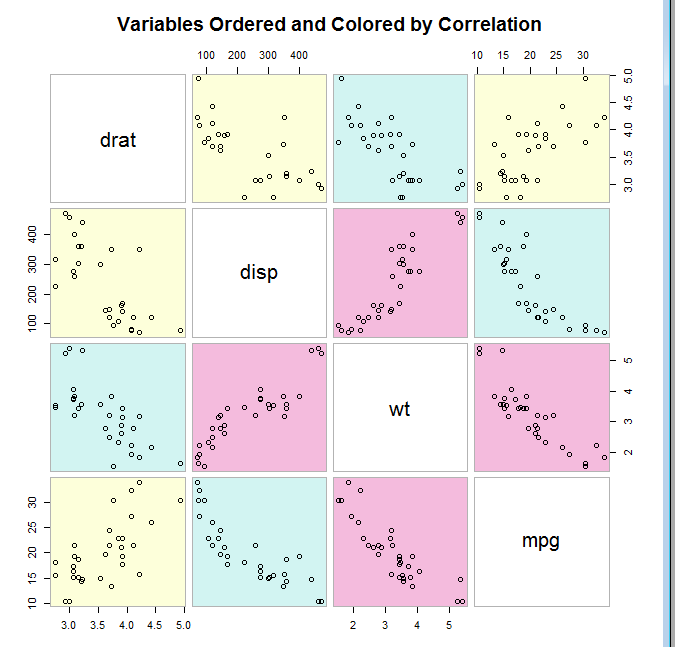3、高密度散点图 当数据点重叠很严重时，用散点图来观察变量关系就显得“力不从心”了。下面是一个人为设计的例子，其中10 000个观测点分布在两个重叠的数据群中： 创建10000个观测数据 set.seed(1234) n <- 10000 c1 <- matrix(rnorm(n, mean=0, sd=.5), ncol=2) c2 <- matrix(rnorm(n, mean=3, sd=2), ncol=2) mydata <- rbind(c1, c2) mydata <- as.data.frame(mydata) names(mydata) <- c("x", "y") （1） with(mydata, plot(x, y, pch=19, main="Scatter Plot with 10000 Observations"))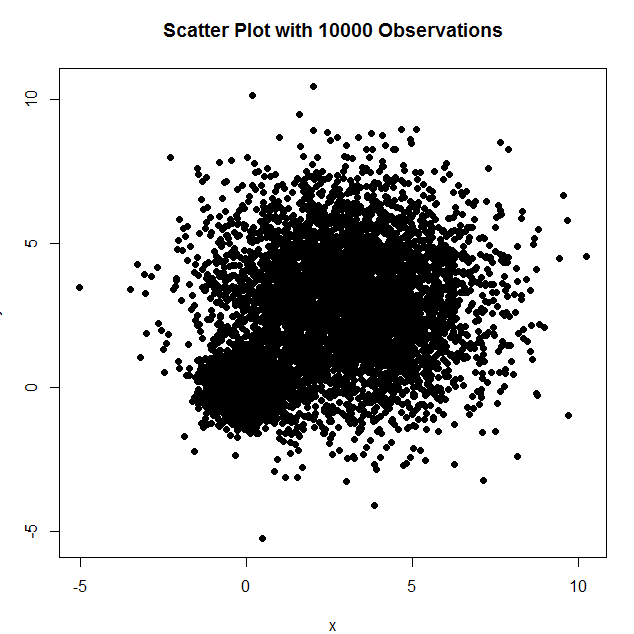2、with(mydata, smoothScatter(x, y, main="Scatterplot colored by Smoothed Densities"))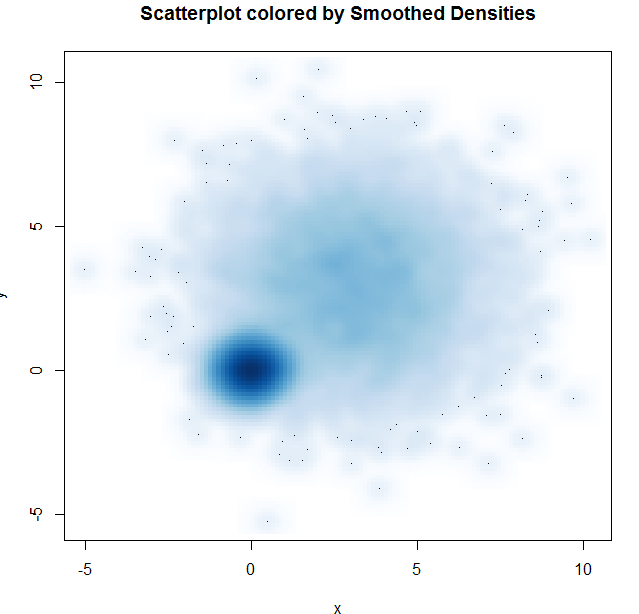3、 library(hexbin) with(mydata, { bin <- hexbin(x, y, xbins=50) plot(bin, main="Hexagonal Binning with 10,000 Observations") })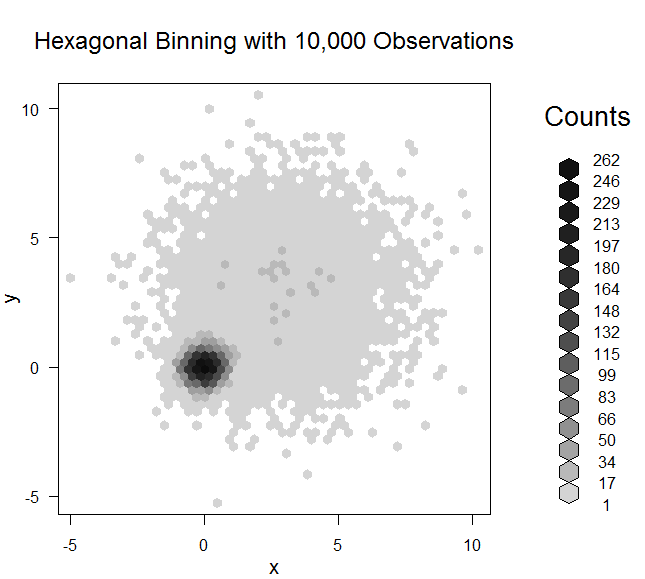4、三维散点图 library(scatterplot3d) attach(mtcars) scatterplot3d(wt, disp, mpg, main="Basic 3D Scatterplot")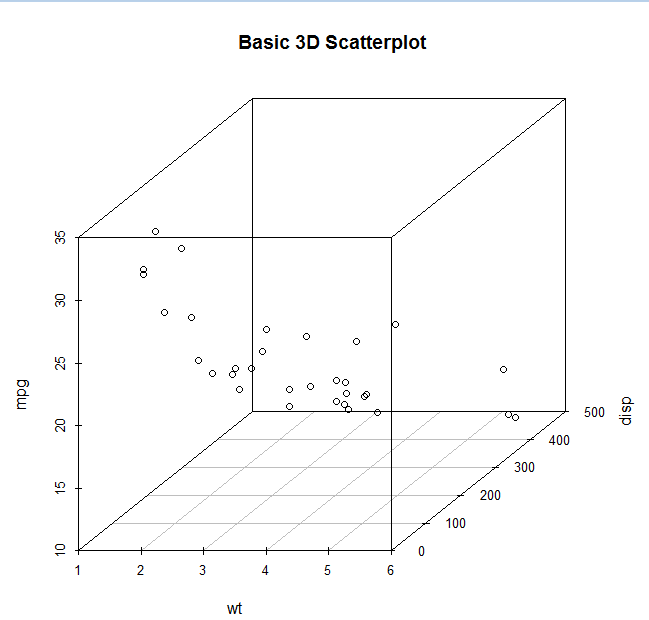s3d <-scatterplot3d(wt, disp, mpg, + pch=16, + highlight.3d=TRUE, + type="h", + main="3D Scatter Plot with Verical Lines and Regression Plane") > fit <- lm(mpg ~ wt+disp) > s3d$plane3d(fit) > detach(mtcars)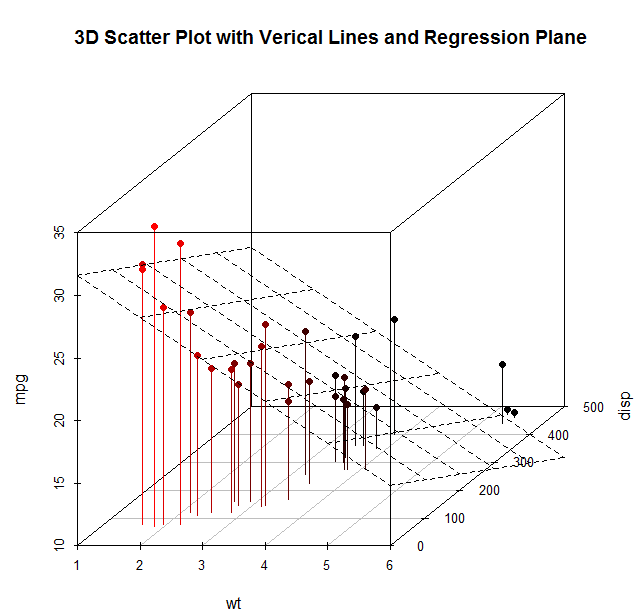这个三维图可以旋转
library(rgl) attach(mtcars) plot3d(wt, disp, mpg, col="red", size=5)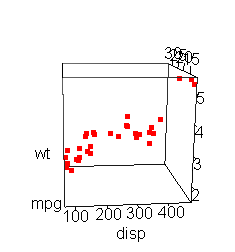4、气泡图
我们通过三维散点图来展示三个定量变量间的关系。现在介绍另外一种思路：先创建一个二维散点图，然后用点的大小来代表第三个变量的值。这便是气泡图（bubble plot）
attach(mtcars) r <- sqrt(disp/pi) symbols(wt, mpg, r, inches=0.30, fg="white", bg="lightblue", main="Bubble Plot with point size proportional to displacement", ylab="Miles Per Gallon", xlab="Weight of Car (lbs/1000)") text(wt, mpg, rownames(mtcars), cex=0.6) detach(mtcars) par(opar)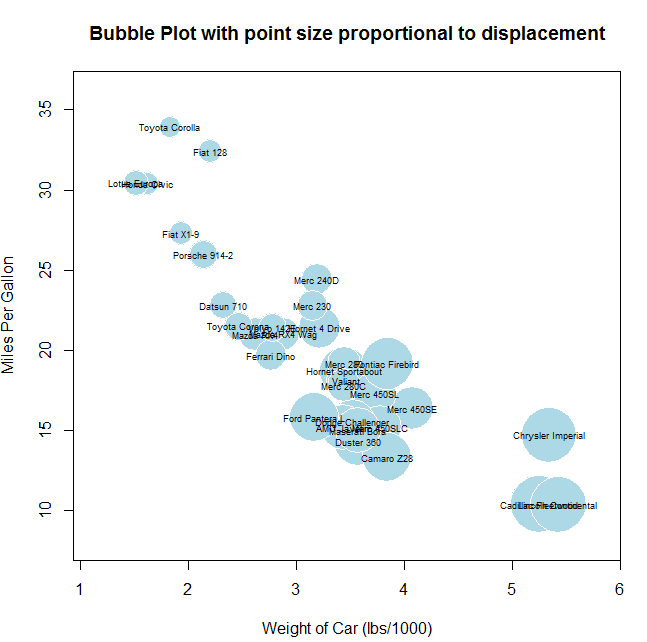5、折线图
par(mfrow=c(1,2)) t1 <- subset(Orange, Tree==1) plot(t1$age, t1$circumference,     xlab="Age (days)",      ylab="Circumference (mm)",      main="Orange Tree 1 Growth") plot(t1$age, t1$circumference,     xlab="Age (days)",     ylab="Circumference (mm)",     main="Orange Tree 1 Growth",      type="b") par(opar)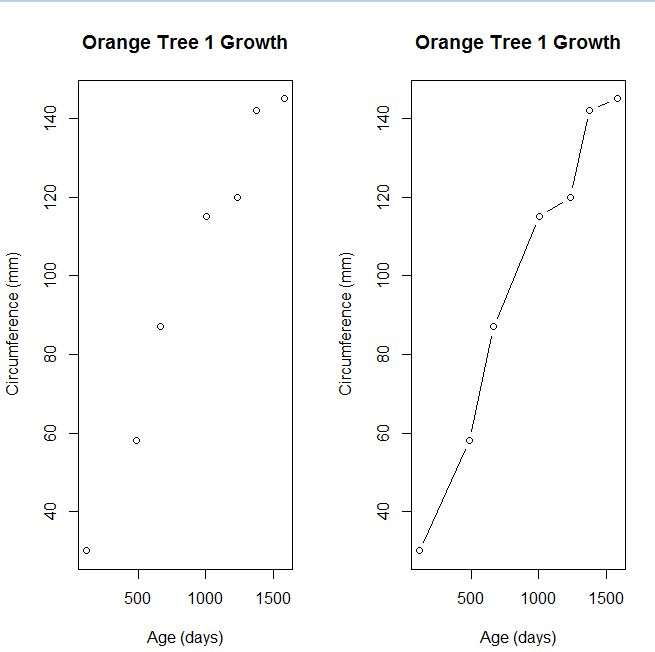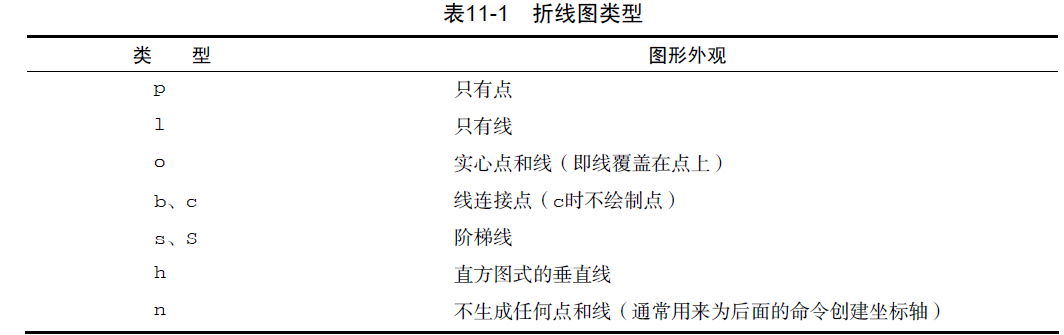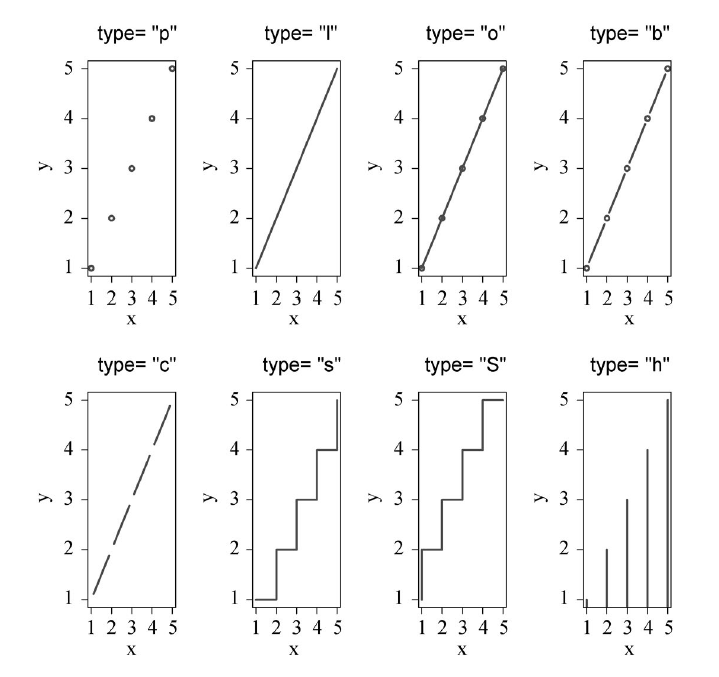Orange$Tree <- as.numeric(Orange$Tree)       ntrees <- max(Orange$Tree) xrange <- range(Orange$age)                  yrange <- range(Orange$circumference) plot(xrange, yrange, type="n", xlab="Age (days)", ylab="Circumference (mm)" ) colors <- rainbow(ntrees) linetype <- c(1:ntrees) plotchar <- seq(18, 18+ntrees, 1) for (i in 1:ntrees) { tree <- subset(Orange, Tree==i) lines(tree$age, tree$circumference, type="b", lwd=2, lty=linetype[i], col=colors[i], pch=plotchar[i] ) } title("Tree Growth", "example of line plot") legend(xrange, yrange, 1:ntrees, cex=0.8, col=colors, pch=plotchar, lty=linetype, title="Tree" )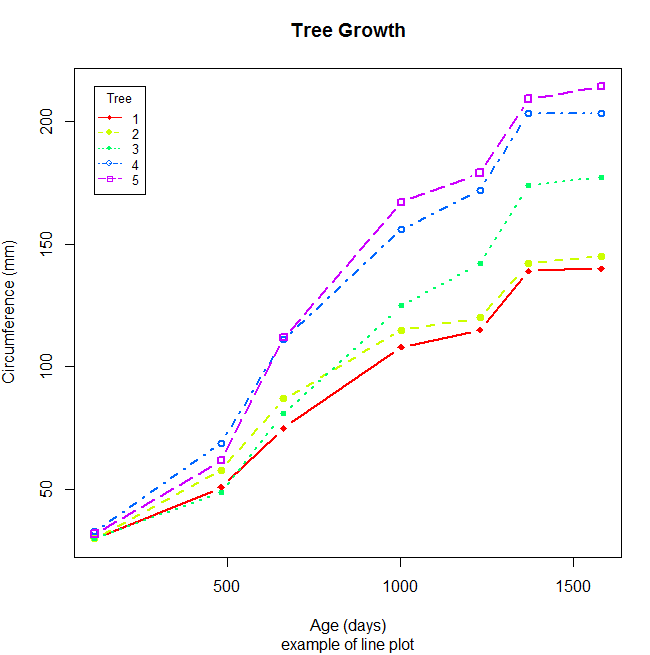展开全文• R语言基础教程1：数据类型R语言基础教程2：散点图R语言基础教程3：曲线图、误差线和图例R语言基础教程4：柱形图R语言基础教程5：图形页面排版R语言基础教程6：程序设计基础R语言基础教程7：数据描述性统计先了解一个...  先了解一个概念：函数。简单地说，把一些R语句（赋值、计算或其他操作步骤）包装起来并给它一个名称，这就是函数。我们前面接触过的getClass( ), class( ), head( ), rep( ), cbind( ), rbind( )等都是函数。显示（打印）对象也有函数print( )，但R有更简单的方法：输入对象名（数据对象或函数名）就可以显示其内容。函数一般都有参数。 R作图也是通过函数完成的，不同函数可以做出不同的图形，比如plot()函数可以做散点图、线状图，barplot()函数可以做出柱状图或条形图，pie()函数用于做饼形图。作图或者称数据可视化，是R的主要的功能之一。R语言虽然是一种计算机语言，但用R作图不一定要会编程，相当简单。 散点图是最基本的数据分析图形，我们就从它开始。 一、文件数据读取和保存 作图首先要有数据。为避免重复输入，我们一般将R使用的数据存成文本文件（txt文件）或逗号分隔符文件（csv文件），Excel里面有这样的保存选项。 好的R软件包一般都提供有一些供学习和举例用的样本数据，这些数据可以用data( )函数来查询。为方便说明，我们使用R（base包）提供的样本数据。先看看有些什么： > data() Data sets in package 'datasets': AirPassengers Monthly Airline Passenger Numbers 1949-1960 BJsales Sales Data with Leading Indicator BJsales.lead (BJsales) Sales Data with Leading Indicator BOD Biochemical Oxygen Demand CO2 Carbon Dioxide Uptake in Grass Plants ChickWeight Weight versus age of chicks on different diets DNase Elisa assay of DNase #...........（还有很多，节省篇幅不列了） 我们用trees数据来做散点图。先看看它是什么样的数据： > str(trees) #数据的结构 'data.frame': 31 obs. of 3 variables:$ Girth : num  8.3 8.6 8.8 10.5 10.7 10.8 11 11 11.1 11.2 ...
$Height: num 70 65 63 72 81 83 66 75 80 75 ...$ Volume: num  10.3 10.3 10.2 16.4 18.8 19.7 15.6 18.2 22.6 19.9 ...

Girth Height Volume
1   8.3     70   10.3
2   8.6     65   10.3
3   8.8     63   10.2
4  10.5     72   16.4
5  10.7     81   18.8
6  10.8     83   19.7
可以看到trees数据集是我们比较熟悉的数据框类型，有3个列变量。我们把它保存到D盘根目录。首先用setwd( )函数设置工作目录，它是R读取和保存文件的默认位置。注意：如果是反斜杠得用两个。下面两条语句效果都一样：
> setwd("d:/")> setwd("d:\\")
然后用write.csv( )函数保存成trees.csv文件：
> write.csv(trees, "trees.csv")
再看看读入的数据是什么样子：
> str(the.data)
'data.frame':    31 obs. of  4 variables:
$X : int 1 2 3 4 5 6 7 8 9 10 ...$ Girth : num  8.3 8.6 8.8 10.5 10.7 10.8 11 11 11.1 11.2 ...
$Height: int 70 65 63 72 81 83 66 75 80 75 ...$ Volume: num  10.3 10.3 10.2 16.4 18.8 19.7 15.6 18.2 22.6 19.9 ...
X Girth Height Volume
1 1   8.3     70   10.3
2 2   8.6     65   10.3
3 3   8.8     63   10.2
4 4  10.5     72   16.4
5 5  10.7     81   18.8
6 6  10.8     83   19.7
> the.data <- the.data[ ,2:ncol(the.data)]
二、快速作图法
用plot函数可以非常便捷地做出散点图：
> x <- the.data$Girth > y <- the.data$Height
> plot(x, y, main = "树围与树高关系图", xlab = "Girth", ylab = "Height")R的快速作图方式主要是针对数据关系的探索性分析的，不同类型的图完全可以显示出数据间的关系，同时避免了浪费时间在图形外观的设置上。如果想美化图形，也很容易。图形元素无非包括标题、坐标轴、点、线、图例等几个部分，外观包括形状、颜色、大小、宽度和字体等。
1、标题文字设置参数：main（主标题），sub（副标题），xlab（x轴标题）和ylab（y轴标题）
2、坐标轴有无：axes （TRUE/FALSE）
3、坐标轴区间参数：xlim，ylim
4、点类型参数：pch
5、线类型、宽度参数：lty，lwd
6、颜色设置参数：col, col.axis, col.lab, col.main, col.sub
7、大小（包括图形和字体）参数：cex, cex.axis, cex.main, cex.sub, cex.lab
8、如果点类型是空心点（pch=21-25），还可以设置内部填充颜色：bg参数
我们通过设置plot函数的参数做一个散点图：
> plot(x, y, main = "树围与树高关系图", xlab = "Girth", ylab = "Height",
xlim = c(0,25),                             #设置x轴刻度范围
pch = 2, col = "red", cex = 1.2,            #设置图形几何形状和颜色
col.lab = "blue", col.axis = "orange",
cex.lab = 1.2, cex.main = 1.5)为了避免输入错误后重复打字，一般先把绘图语句写到文本文件，然后拷贝到R里面就可以了。有关的文本编辑器后面再推荐和介绍，现在可以先用自己熟悉的任何文本编辑器做这个事情。
三、分步作图法
上面的图形可以一步步进行。
1、先绘制只设置了点的图，轴不显示，坐标标题为空：
> plot(x, y, pch = 2, col = "red", axes =FALSE, xlab="", ylab="")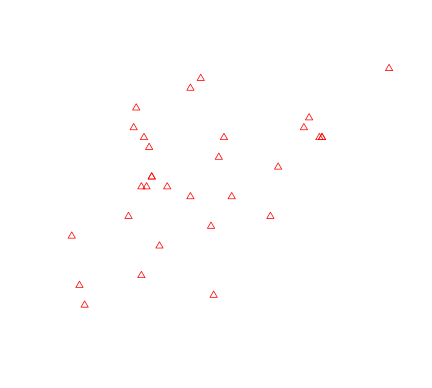2、添加其他图形元素（注意每执行一条语句后图形会马上有变化，中间过程的图形就不一一贴出了）：

> plot(x, y, pch = 6, col = "red", axes =FALSE, xlab="", ylab="", xlim=c(8,22), ylim=c(10,90))
> box(col="green")
> axis(1, col="orange", lty=2, lwd=3)
> axis(2, col="purple", col.axis="purple")
> axis(4, col="seagreen")
> title(main="树围与树高关系图", cex.main = 1.5)
> title(xlab="Girth", cex.lab=1.2, col.lab="blue")可以看到，分步作图法可以获得对图形元素的更多控制，各个坐标轴、标签的颜色和字体大小都可以单独设置。还可以在上面图形的基础上添加其他的数据点（在前面作图时注意设置y轴的范围）：
> y2 <- the.data\$Volume
> points(x, y2, pch=25, col="red", bg="blue")
> title(ylab="Height or Volume", cex.lab=1.2, col.lab="red")注意：plot( )函数执行后会把图形区的其他东西全“擦”掉再新作图，这样的图形函数称为高水平图形函数；而box( ), title( ), axis( ), points( )这些函数只是在原有图形基础上添加东西，它们称为低水平图形函数。只有使用了高级图形函数产生图形区后才能使用低水平图形函数。各图形元素的低水平图形函数有：
1、添加标题（主、副标题和坐标轴标题）：title( )
2、添加坐标轴：axis( )
3、添加数据点：points( )
4、添加线：lines( )
5、添加水平线或垂直线：abline( )
6、其他text( ), polygon( ), legend( )在后面介绍。

四、R base函数作图的特点
以上方法是用都是R基本绘图包（graphics包，已包含在R基本安装包里面）带的作图函数来做的，这个过程就像拿起画笔在一张白纸上画画，每个图形元素都可以自己去设计。因此R graphics包作图的特点也就体现出来了：R提供了丰富的图元控制函数和参数，可以方便地描绘出所需的每个元素。但是和我们普通作图不一样的是，R作图没有橡皮擦，做错了只能重新来过。但这应该不是什么问题，R作图是用语句来进行的，重用这些语句可以绘制出完全一样的图，这点正是科学绘图所需要的。
但是，R把图形元素的控制全交给了我们，虽然很自由，是不是也很麻烦？这恐怕也是很多人学R作图过程中退却的原因吧？但这些设置是有懒办法的：把常用的作图参数设置好并作为函数封装起来（类似与一些软件的宏操作），这样就可以一劳永逸了。这是后话。

展开全文• 直方 实例：nutshell包的births2006.smpl数据集，包含了2006年美国出生人口的数据的10%样本，每一条记录有13个变量。使用数据集前，需通过install.packages(“nutshell”)安装并加载。 以美国出生人口数据为例，...
• 支持Circos 2D数据轨道绘图，例如散点图、线、柱状图、连接器等 实现： library(RCircos) library(Cairo) outF&lt;-'/Output/Circos.pdf' data(UCSC.HG19.Human.CytoBandIdeogram) data(RCircos.Scatter....
• plot()是R中为对象作图的一个泛型函数（它的输出将根据所绘制对象类型的不同而变化）；plot(x,y,type="b")表示将x置于横轴，y置于纵轴，绘制点集（x，y），然后使用线段将其连接；type="b"表示同时绘制点和线，使用...
• ## R语言ggplot2绘制多条折线图

万次阅读 多人点赞 2020-10-22 22:12:28
涉及ggplot2使用知识点：去掉背景色及网格线，保留坐标轴边框；设置显示中文字体；更改图例位置至内并添加边框线使图片更美观；设置坐标轴刻度值。除此之外，还有数据的融合处理。
• R语言实战笔记–第十六章 高级图形进阶标签（空格分隔）： R语言 lattice 交互式图形图形系统简介　本文为R语言实战中最后一章，介绍的是图形的高级进阶，主要讲述了两个包（lattice和ggplot2）以及交互式图形的做法...lattice
• 写在前面：为啥不用excel绘制这些，用PoweBI，帆软BI等可视化软件来绘图，不是更方便吗？的确，这些工具都很方便，但同时，它们显得很呆，不够灵活，更为致命的是，它们绘制出的图形，分辨率不够，用来出版论文，...
• R语言基础图形分为创建图形与添加图形内容。 创建图形 条形图 饼图 直方图 核密度图 箱线图 点图 散点图 折线图 相关图 马赛克图 添加图形内容 图形参数：符号、线条、颜色、文本属性、图形尺寸与边界尺寸 标题...可视化
• plot(x)  以x的元素值为纵坐标、以序号为横坐标绘图 plot(x,y)  x(在x-轴上)与y(在y-轴上)的二元作图 sunflowerplot(x,y)同上，...boxplot(x)盒形(“box-and-whiskers”) stripchart(x)把x的值画在一条线段上
• ## R语言绘图基础

千人学习 2017-02-18 21:49:00
介绍R语言的绘图基础，如固有颜色、RGB取色、主题调色板介绍，文字字体、颜色、大小等参数详解，点样式、颜色、大小等参数详解，线条样式、颜色、粗细等参数详解；详细介绍R低级绘图函数：标题、图例、坐标轴、网格...
• 高级绘图-气泡 数据准备 颜色准备 散点大小 绘制图形 高级绘图-曲线 数据准备 颜色准备 画图 高级画图-柱状 数据准备 颜色配置 画图 高级画图-条形 数据准备 画图 数轴上的标签 高级画图-饼图 高级...
• 散点图用于描述两个连续性变量间的关系，三个变量之间的关系可以通过3D图形或气泡来展示，多个变量之间的两两关系可以通过散点图矩阵来展示。 一，添加了最佳拟合曲线的散点图 使用基础函数plot(x,y)来绘制散点图...
• R语言问题讨论交流，...R语言作为顶级的可视化编程语言，以其出色的绘图功能闻名于统计届。 画图命令可以被分成三种基本类型： （1）高级绘图函数：把数据作为函数的参数来产生一个图形。所有中标签均由函...Mac 可视化 数据挖掘
• 高级绘图工具是相对于R的基础绘图系统而言的，包括lattice图形系统、ggplot2图形系统以及各类交互式绘图系统。 lattice包是由Deepayan Sarkar基于Grid包编写的一套统计图形系统，他的图形设计理念来自于Cleveland的...
• 】小编在介绍了R语言基础入门(包括R的安装和载入、数据导入和导出方法等)，在这一期小编继续为你挖掘R语言的巧妙用处，跟大家探讨如何创建和保存图形，如何修改图形中的特征如标题、坐标轴等。Part 1创建和保存图形R...
• 【小白福利】从零开始学习R语言（一）——数据结构之“向量（Vector）：Nick WU：【小白福利】从零开始学习R语言（一）——数据结构之“向量”​zhuanlan.zhihu.com【小白福利】从零开始学习R语言（二）——数据结构...
• #ggplot2 ...#散点图 ggplot(data=mtcars,aes(x=wt,y=mpg))+geom_point()+labs(title="Automation Data",x="Weight",y="Miles Per Gallon") #散点图+回归曲线 ggplot(data=mtcars,aes(x=wt,y=...
• R语言常见图形汇总，使用R默认的高级函数和ggplot2等多个包数据分析 数据挖掘
• 不同图表的实现-R语言ggplot2基础教程 一个有效的图表是： 传达正确的信息、不扭曲事实。 简单而优雅。 凸显特征，而不是掩盖信息。 不会信息过载（元素过多）。 下面的列表根据其主要目的对可视化方法进行了分类...可视化 数据分析
• R语言小白学习笔记4—统计图笔记链接学习笔记4—统计图4.1 基础统计图4.1.1基础直方图4.1.2基础散点图4.1.3箱线图4.2 ggplot24.2.1 ggplot2:直方图和核密度曲线4.2.2 ggplot2:散点图4.2.3：箱线图和小提琴图4.2.4 ...大数据...